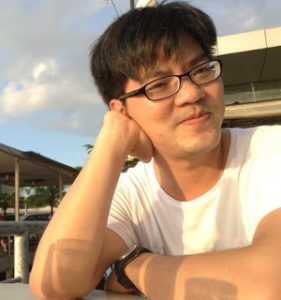# Trần Vũ Khanh Assoc. Prof. PhD

PhD in Math (2010), University of Padova, Italy

Prof. Tran Vu Khanh completed his Ph.D. in Mathematics at the University of Padova (Italy, 2010). After that, he worked at our Tan Tao University (2011), the National University of Singapore (Singapore, 2013) and the University of Wollongong (Australia, 2015). His research papers  were published in high prestige journals such as Inventiones Mathematicae, Advances in Mathematics. Prof. Khanh was awarded the 2011 Scientific Prize from the Institute of Mathematics in Hanoi, Vietnam for his particularly outstanding achievements in mathematics. In 2013, he was invited to be one of five plenary speakers in the largest mathematical conference in Vietnam. He completed the national research project of Australia (ARC DECRA 2019) and of Vietnam (NAFOSTED 2014). Currently, he is a member in editorial board of Vietnam Journal of Mathematics, Journal of Korean Mathematical Society (a SCI journal).

His major research interests include:

• Several Complex Variables
• Inverse Problem
• Data Science, Machine Learning, Artificial Intelligence

The recent scientific publications:

1. (with Jiakun Liu, Phung Trong Thuc) Bergman-Toeplitz operators on fat Hartogs triangles, Proc. Amer. Math. Soc, (2019), 327-338.
2. (with Jiakun Liu, Phung Trong Thuc) Bergman–Toeplitz operators on weakly pseudoconvex domains, Math. Z. (2019), 591-607.
3. (with Luca Baracco, Stefano Pinton) The complex Monge-Amp\`ere equation on weakly pseudoconvex domainsComptes Rendus Math. 355 (2017), 411-414.
4. (with Nhat-Tan Le, Duy-Minh Dang) A Fourier Sine transform decomposition approach for valuing American knock-out options with time-dependent rebates J. Comput. Appl. Math. 317 (2017), 652-671.
5. (with Luca Baracco, Stefano Pinton and Giuseppe Zampieri) Holder regularity of the solution to the complex Monge-Amp`ere equation with L^p densityCalc. Val. Partial Differential Equations 55 (2016), 55:74
6. (with Ninh Van Thu) Iterates of holomorphic self-maps on pseudoconvex domains of finite and infinite type in \$C^n\$, Proc. Amer. Math. Soc.  144 (2016),  5197–5206.
7. Equivalence of estimates on domain and its boundary, Vietnam J. Math . 44 (2016), 29-48.
8. Lower bounds on the Kobayashi metric near a point of infinite type, J. Geom. Anal. 26 (2016), 616–629.
9. (with Stefanno Pinton and Giuseppe Zampieri) Loss of derivatives in the infinite type, Pure Appl. Math. Q. 11 (2015) , 315–327.
10. (with Ly Kim Ha) Boundary regularity for the Complex Monge-Amp`ere equation on peudoconvex domains of infinite typeMath. Res. Lett. 22, (2015), 467–484.
11. (with Luca Baracco and Giuseppe Zampieri) Hypoellipticity of the D-bar-Neumann problem at exponentially degenerate points, Asian J. Math. 18 (2014), 623-632.
12. (with Ly Kim Ha and Andrew Raich) L^p-Estimates for the D-bar-equation on a class of infinite type domains, Internat. J. Math. 25 (2014), 1450106 [15 pages].
13. (with Giuseppe Zampieri) Precise subelliptic estimates for a class of special domains,  J. Anal. Math. 123 (2014), 171–181.
14. Lower bound for the geometric type from a generalized estimate in the D-bar-Neumann problem – a new approach by peak functions, Michigan Math. J. 63 (2014), 209–212.
15. (with Giuseppe Zampieri) Regularity at the boundary and tangential regularity of solutions of the Cauchy-Riemann system, Pacific J. Math. 265 (2013), 491–498.
16. Supnorm and f-H\”older estimates for D-bar on convex domains of general type in C^2, J. Math. Anal. Appl. 403 (2013), 522–531.

See more here